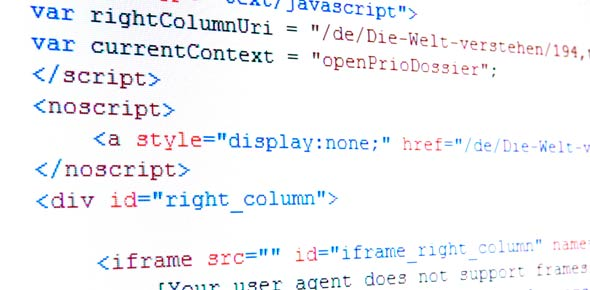# Can You Pass This C Language Coding Test?

10 Questions | Attempts: 11016SettingsC is a programming language which was developed by Dennis Ritchie. C language incorporates low-level access to memory, clean style and simple set of keywords which makes 'C' language a perfect system programming like Operating system. This quiz has been designed to test your programming skills and basic knowledge about C language. There is a set of 10 questions. Read the questions carefully and answer. So, let's try out the quiz. All the best!

• 1.
Void main() { int const * p=5; printf("%d",++(*p)); }
• A.

COMPILER ERROR

• B.

6

• C.

• D.

5

• E.

NONE

• 2.
Main() { char s[ ]="man"; int i; for(i=0;s[ i ];i++) printf("\n%c%c%c%c",s[ i ],*(s+i),*(i+s),i[s]); }
• A.

Mmm aaaa nnnnn

• B.

Mmmmm aaa nnnn

• C.

Mmmm aaaa nnnn

• D.

Mmm aaa nnn

• E.

NONE

• 3.
6. main() { extern int i; i=20; printf("%d",i); }
• A.

• B.

COMPILER ERROR

• C.

20

• D.

• E.

NONE

• 4.
Predict the output of the following C snippets int main() {     int main = 56;     printf("%d", main);     return 0; }
• A.

Compiler Error

• B.

Depends on the compiler

• C.

56

• D.

None of above

• 5.
What is the output of this C code? #include int main() {    float a = 3.14, *fptr;    fptr = &a;      printf("Size of Float Pointer : %d", sizeof(fptr));    return (0); }
• A.

Size of Float Pointer : 2

• B.

Size of Float Pointer : 4

• C.

Size of Float Pointer : 6

• D.

Size of Float Pointer : 8

• 6.
What is the output of the below c code? #include<stdio.h> int main() {     char ch;     if(ch = printf(""))         printf("It matters\n");     else         printf("It doesn't matters\n");     return 0; }
• A.

It matters

• B.

It doesn’t matters

• C.

Run time error

• D.

Nothing

• 7.
Which one do you like? int main() {     fork();     fork();     printf("Hello world\n"); }
• A.

1

• B.

2

• C.

4

• D.

8

• 8.
What is the output of this C code? void main() {     int i, j;     for(i=0,j=0;i<10,j<20;i++,j++){         printf("i=%d \t j=%d\n", i, j);     } }
• A.

Print i and j till 19

• B.

Print i till 9 and j till 19

• C.

Print i and j till 9

• D.

Runtime error

• 9.
What is the output of this C code? #include<stdio.h> int main() {     int x=1, y=0,z=5;     int a=x&&y||++z;     printf("%d",z++); }
• A.

1

• B.

5

• C.

6

• D.

7

• 10.
What is the output of this C code? #include<stdio.h> int main() {     int y = 2;     int z = y +(y = 10);     printf("%d\n", z); }
• A.

2

• B.

4

• C.

20

• D.

Compile error

## Related TopicsBack to top
×

Wait!
Here's an interesting quiz for you.